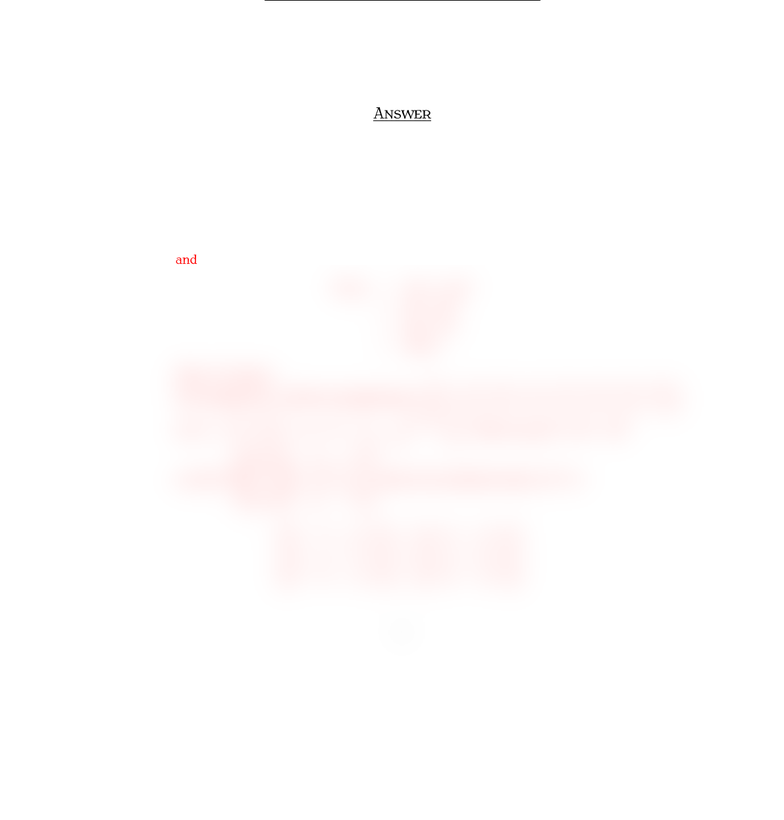# Quiz11Spr11mt210Sample1Ans

33 views2 pagesMT Q  S 
İ S. Y
A , 
Surname, Name:
Q . §. C S
Deﬁne T:M2×2ÏM2×2by T(A) = AAt.
(a) Show that Tis a linear transformation.
(b) Find a basis for the range of T
(c) Find a basis for the kernel of T.
(d) Verify that the Rank Theorem holds.
A
(a) Note that
T(A+B) = (A+B)(A+B)t
=A+BAtBt
=AAt+BBt
=T(A) + T(B).
and
T(cA) = (cA)(cA)t
=cA cAt
=c(AAt)
=cT(A).
Thus, Tis linear.
(b) Consider M2×2with the standard basis: {[ 1 0
0 0 ],[0 1
0 0 ],[0 0
1 0 ],[0 0
0 1 ]}.
Let A=[a b
c d ]ÑAAt=[0bc
cb0]. Then we get T:R4ÏR4
so that T
a
b
c
d
=
0
bc
cb
0
where the standard matrix of Tis
0 0 0 0
0 1 1 0
01 1 0
0 0 0 0
0 0 0 0
0 1 1 0
0 0 0 0
0 0 0 0
.
1
Unlock document

This preview shows half of the first page of the document.
Unlock all 2 pages and 3 million more documents.

## Document Summary

T(a + b) = (a + b) (a + b)t. = a at + b bt. Thus, t is linear. (b) consider m2 2 with the standard basis: {[ 1 0. Let a = [ a b c d ] a at = [ so that t . 0 b c a b c d. Then we get t : r4 r4. 0 1 ]}. c b where the standard matrix of t is. The range of t is given as x2 which implies that. : x2 r range of t = {x2[ 1 0 ] : x2 r} . 1 0 ]} is a basis for the range of t. Thus, the set {[ (c) note that the null space of t is null t = implies that kernel of t = {x1[ 1 0. 0 1 ] : x1, x2, x4 r} .